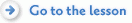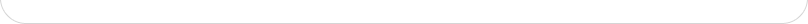﻿ Spicynodes : Negatives (Math) - Background

# Site archive. SpicyNodes was active 2005-2018.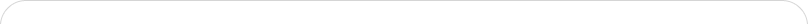The idea of a negative number emerged in China around 200 BCE. Like many mathematical concepts, negative integers emerged from accounting. Many people are familiar with the term "in the red" if they owe money, and "in the black" if they have money. These terms actually come from ancient Chinese accounting methods, where red rods were used to represent a debt, while black rods were used to represent a surplus. If you had the same number of red rods as you had black rods, you were breaking even.

An integer is simply a whole number or zero, and includes positive numbers and negative numbers. Many students find the concept of negative numbers hard to grasp. After all, if the nature of numbers is to denote quantity, how can you have a negative quantity? Although it’s true that you can’t have -5 apples in the refrigerator, when you consider the foundation of negative numbers, it is eminently possible to owe 5 apples. You can, for example, think, "I ate all 5 of the apples in the fridge. I need to go to the store and buy 5 more to replace them," and then represent that deficit as -5. Negative numbers can also symbolize direction. For example, if North is considered positive, and South is considered negative, you could use this designation to calculate movement. If I walk 3 meters North (positive 3), then turn around and walk South 6 meters (negative 6), what is my final position with respect to my original position (net movement or displacement)? 3 + (-6) = -3, or 3 meters South of my original position.

Once students understand that negative and positive symbols can denote opposites such as direction (up/down, right/left, east/west), temperature (hot/cold), operations (add/remove), they will be able to grasp the concepts and processes of integer operations beyond the formal rules that they see in text books.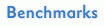This lesson plan addresses topics covered in General Curriculum Outcome (GCO) B in the Nova Scotia grade 7 math curriculum, as well as the National Council of Teachers of Mathematics (NCTM) standards and all the USA State Standards for secondary math education.

General Curriculum Outcome (GCO) B
B11: Add and subtract integers concretely, pictorially, and symbolically to solve problems
B12: Multiply integers concretely, pictorially, and symbolically to solve problems
B13: Divide integers concretely, pictorially, and symbolically to solve problems
B14: Solve and pose problems which utilize addition, subtraction, multiplication, and division of integers.

NCTM Standards ( Number and Operations Standard for Grades 6–8)
In grades 6-8 all students should

* develop meaning for and represent integers and compare quantities with them.
* understand the meaning and effects of arithmetic operations with fractions, decimals, and integers
* develop and analyze algorithms for computing with fractions, decimals, and integers and develop fluency in their use.

In addition, SpicyNodes provides the opportunity to students to meet with the NCTM standards for communication, connections, and representation.

 Instructional programs from pre-K through grade 12 should enable all students to * create and use representations to organize, record, and communicate mathematical ideas; * organize and consolidate their mathematical thinking through communication; * recognize and use connections among mathematical ideas; * communicate their mathematical thinking coherently and clearly to peers, teachers, and others; * analyze and evaluate the mathematical thinking and strategies of others; * use the language of mathematics to express mathematical ideas precisely.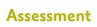See ideas for assessing this lesson, and also a you can adapt. Also, for the content of this lesson, you can also use the rubric below.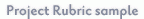4321
Integer concepts coverage and analysis – demonstrated by SpicyNode label descriptions, definitions and connectionsExcellent understanding of negative integers and arithmetic operations.

Provides examples for all the operations.

Presents multidisciplinary connections
Good understanding of negative integers and arithmetic operations.

Provides examples for all the operations.
Satisfactory understanding of negative integers and arithmetic operations.

Examples may be missing or some may be inaccurate
Little understanding of negative integers and arithmetic operations.
CommunicationVery Effective organization and presentation of ideasEffective organization and presentation of ideasSatisfactory organization and presentation of ideasInaccurate organization and presentation of ideas
CollaborationSelf-directed and shared responsibilitiesSelf-directed and shared responsibilitiesShared responsibilitiesNeeded teacher’s help and intervention with task delegation1. Understanding a concept from the ground up is essential when teaching ideas that are abstract, yet fundamental. A good book that explains the basics is Mathematics for Elementary Teachers: A Contemporary Approach, 7th Edition, which can be purchased at site: Wiley
1. Visual Math learning site for integer arithmetic: Integers1. Eves, Howard. An Introduction to the History of Mathematics, Sixth Ed. Brooks/Cole: Thomson Learning. 1990
1. Struik, Dirk. A Concise History of Mathematics. New York: Dover Publications Inc. 1967.
1. There are very few complete math history resources available, and most are outdated and plagiarize directly from each other. However, St. Andrews University in Scotland has a great site that can be found at The MacTutor History of Mathematics archive
1. Leo Rogers has written an article that is specific to negative numbers, and that has excellent historical examples: The History of Negative Numbers